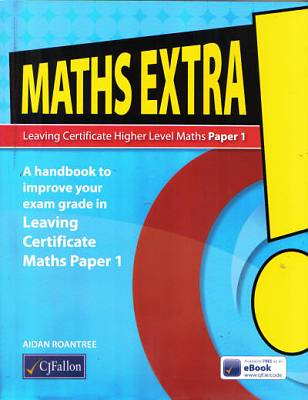# Maths Extra! Leaving Certificate Higher Level Maths Paper 1

Welcome to Maths Extra! Higher Paper 1. This book is a concise, exam-focused handbook for the Leaving Certificate Maths, Paper 1 exam. It contains all the information you will need in your preparation for this exam.

You can use Maths Extra! Higher Paper 1 in conjunction with your textbook, as you make your way through the maths course. It will help you to read, plan, time and answer your exam questions in the most efficient and effective manner.

This book summarises all the key concepts, formulae and methods in each of the topic areas that is covered by Paper 1. They are presented in an accessible, clear format where much of the long backgrounds, already covered in your textbook, has been omitted or briefly covered.

It is divided into chapters where all the connected ideas are dealt with together. For example, Chapter 2, called Solving Equations by Algebra, covers, in one location, the methods that can be used to solve any kind of equation that you can possibly meet in your exam.

Starting with linear and quadratic equations, it moves on rapidly to the various types of simultaneous equations, cubic equations, rational and irrational equations. It also deals with equations with the unknown in the index, log equations and modulus equations. By the end of this chapter, any method of solving an equation by algebra has been covered.

In most textbooks, these topics are spread over many chapters. The fact that all these methods are gathered together should be of invaluable benefit to students for whom choosing a method to solve a given equation is an issue, and as a general reference for all students.

Many other chapters have a similar objective, and are organised in a similar fashion.

To reflect exam questions, the use of graphs has been given an extensive treatment through a number of chapters. Also, as in exam questions to date, there are numerous examples which require methods from a number of different topic areas

ISBN: 9780714423821

€ 10.45

In Stock.

Usually despatched in 2-5 working days.Paperback

Maths Extra! Leaving Certificate Higher Level Maths Paper 1 also appears in these Categories: